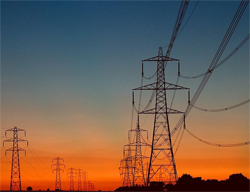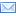#Difference Between Similar Terms and Objects

# Difference Between kVA and kWkVA vs kW

Have you ever noticed that with every appliance or piece of electrical machinery that you avail, they will always indicate their respective power ratings?

You will notice that some electrical equipment express their power ratings in kW, or kilowatts; and some are expressed in kVA, or kilo Volt Amperes. Both values express power, but they are actually different.

kVA is known as the ‘apparent power’ of a particular circuit or electrical system. In direct current circuits, kVA is equal to kW, because voltage and current do not get out of phase. However, ‘apparent power’ and ‘real power’ (which is expressed as kW) may differ in alternating current circuits. kW is simply the amount of actual power that does valid work. It should be noted that only fraction of kVA is accessible to do work, and the rest is an excess in the current.

Solving for the kW (real power) requires another variable called the Power Factor (PF). That so-called Power Factor is a nebulous value that can vary for every appliance or electrical device. In essence, the value of the Power Factor is either given in a percentage, or 0 to 1, wherein 100 percent (or 1) is considered as unity. The closer the Power Factor is to unity, the more efficient a particular device is with its use of electricity.

Unity is practically present in DC circuits, which creates no difference between the kVA and kW. A device uses less kW when the voltage is out of phase with the current. At the same time, the Power Factor naturally lowers in the process. Power Factor will either be leading or lagging, depending on which way the load shifts the phase of the current with respect to the phase of the voltage.

The relationship between the three (kVA, kW, and Power Factor) is mathematically described as:

kW = kVA x Power Factor; kVA = kW / Power Factor; Power Factor = kW / kVA

In DC circuits, the power factor is mathematically inconsequential, because it is in unity. Therefore:

kW = kVA = Volts x Current x 1 = Volts x Current

Summary:

1. kVA is known as the ‘apparent power’, while kW refers to the actual, or real power.

2. kW is the amount of power capable of doing work, while only a portion of kVA is available to do work.

3. kW is kilowatts, while kVA is kilo Volts Amperes.

4. kVA is equal to kW in DC circuits because the voltage and current are not out of phase (unity).

5. However, in AC circuits, voltage and current may get out of phase. Therefore, kW and kVA will differ depending on the Power Factor, or how much leading or lagging occurs.

Latest posts by Ian (see all)

### Search DifferenceBetween.net :

Custom Search

Help us improve. Rate this post!(19 votes, average: 4.26 out of 5)Loading...Email This Post : If you like this article or our site. Please spread the word. Share it with your friends/family.

1. Nice to read your write up on kVA, kW and PF.
I have tried to clear s few ambiguities in this area in my following blog
http://lvnaga.wordpress.com/2010/02/19/electrical-power-and-power-factor/
Nagarajan

2. How much watts can a 1 KVA offline UPS support?

3. is the KW and KVA rating will be same for a single phase generator?

• kva and kw not same in 1 phase bcoz it ac current

4. thanks fo the info on kW and kVA, was searching for it

5. dear sir,
what is the diferance of kva and kw. how to caculate?

• Actually,KVA is appearent power and KW is real that is actual power.Now, in DC circuit KVA is equal to KW.In DC circuits, there is no difference between the kVA and the kW because of the power factor. The power factor leads or lags depending on the way that the load shifts the phase of the current compared to the phase of the voltage. This creates a unity in the DC circuits. In AC circuits, voltage and current may get out of phase leading to a difference in kW and kVA that will be based on the power factor (or how much leading or lagging occurs).

6. Very interesting. I’d always assumed KVA and KW were the same in all circumstances. I consider myself now better educated!!

7. thanks

8. Note with many thanks.

9. Thanks the note author

10. Thanks sir,,,

11. How to calculate the kva and kw

• If a 1kw heater is used for 2 hours then the kW will b 2kwh

1 kw heater
2 hours

1kW *2 hours

So the kW will be 2kwh
N kva will b less depending upon the power factor

• If 1kw is used for 2hrs? Then, if you used for 4hrs you need 2kw

12. THANKS TO LET ME KNOW WHAT IS KVA AND KW.PLEASE,HOW CAN I GET MORE LESSONS?

13. It’s very nice that i get myself for a clear concept. Thank you for shearing such a valuable topic.

Kind regards
Autojit

14. Why used power foctor ?

15. Hi everyone,
I am not from electrical background that is why kindly help me understand one thing.
“Voltage and current do not get out of phase in DC circuits.”
What is the meaning of this phrase here “out of phase”?
I read lots of article but this one helped me in understanding the difference between kw and kva.

• Supposed both current and voltage travelling in same direction and at same angle is called “in phase” if not travelling like that is “out of phase”

16. Thank, what is the difference between kva and kvar?

17. Produce an a expression representing a modern of a fuel coast of a power generation

18. I want detail

19. i m not understanding that USA 160V factor is 60Hz but india 230V factor 50Hz units.
why many different india to USA

• 160volts, 60 Hertz’s
Means the the voltage is 160, and frequency is 60, (which is 60 cycles per second).
That is in alternating current the polarity of the power supply is changed 60 times in a second from positive to negative. In India it is 50 times per second. For more practical perpose we can take it as, the phase line will have its positive polarity and negative polarity alternatily for 50 times in a second. In a filament bulb when it glows we cannot feel the flickering.
Calculation of wattage
In DC supply Watts= Volt X ampear
When a DC motor is running measure the amp in its phase line using a tong tester or amp meter, multiplying it with the supply DC voltage gives the power in watts.
In AC supply the practical methods is same, here watts X power factor gives
the real wattage. In AC machines interaction of the frequency a back EMF is produced the power will not reach the motor in its full effectiveness.
Usually power factor = KVA/watts which in all inductant load will beless than one.
The explanation is purely practical and is just to have some idea about the power supply for practical people.

• thanks for this clarification. I now know the difference between KVA and KW.

20. Thank you sir..

21. I Learn the difference today

22. Great explanation of Kw&KVa

23. Good explain

Please note: comment moderation is enabled and may delay your comment. There is no need to resubmit your comment.

Articles on DifferenceBetween.net are general information, and are not intended to substitute for professional advice. The information is "AS IS", "WITH ALL FAULTS". User assumes all risk of use, damage, or injury. You agree that we have no liability for any damages.

See more about : , ,